###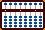Simplifying Subtraction

Using the complementary numbers for 9, here's an efficient and convenient method for solving problems of subtraction. The technique turns these problems around and transforms them into problems of addition.

Terminology: In the problem 3 - 2 = 1,  3 is the minuend, 2 is the subtrahend and 1 is the remainder.

Basically the method is this;

From the subtrahend, take the complementary numbers that make up 9.
Subtract 1 followed by as many zeros as there are numbers in the subtrahend.
Add one to the result to obtain the remainder.

Example 3275 - 689 = 2586

Solving this problem using the conventional subtraction method would require seven moves. Using Fernando's method the problem can be solved in five moves.

Understanding the Complement

**Please note: This is not a step that needs doing each time. I've included it only as a demonstration to show the thinking behind the idea.**

Place the subtrahend 689 on the soroban. This will help us to better identify the complementary numbers that make up 9.  Notice the numbers represented by the grey beads on rods G H & I. When added to its corresponding number in the subtrahend, each of these three numbers will equal 9. On rod G there are 3 grey beads, on rod H there is 1 grey bead and on rod I there are no grey beads for a total of 310. Instead of subtracting in the usual way, add this complementary 310 to the minuend.Fig.1 ```A B C D E F G H I J K . . .     0 0 0 0 0 0 6 8 9 0 0 ```

The Solution

Step 1: Designate rod I to be the unit rod and set the minuend 3275 onto rods FGHI. (Fig.2)Fig.2 ```Step 1 A B C D E F G H I J K . . .     0 0 0 0 0 3 2 7 5 0 0 ```

Step 2:
Since 310 is the complementary number add it to rods GHI. This leaves 3585 on rods FGHI. (Fig.3)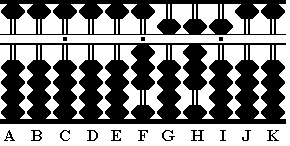Fig.3 ```Step 2 A B C D E F G H I J K . . .     0 0 0 0 0 3 2 7 5 0 0 + 3 1 0 Step 2 0 0 0 0 0 3 5 8 5 0 0 ```

Step 3: Notice the subtrahend. It has three numbers. Subtract 1 followed by three zeros, one zero for each of the three numbers in the subtrahend. Subtract 1000 from rods FGHI.

3a & the answer: Add 1 to the result by adding 1 to rod I. This leaves the remainder 2586 on rods FGHI which is the answer to the problem. (Fig.4)Fig.4 ```Step 3 A B C D E F G H I J K . . .     0 0 0 0 0 3 5 8 5 0 0 - 1 0 0 0 Step 3 0 0 0 0 0 2 5 8 5 0 0 + 1 Step 3a 0 0 0 0 0 2 5 8 6 0 0```

### Life Situations

Here's another example that shows a very good use for this technique. It's a problem that was introduced to the Yahoo Soroban/Abacus  newsgroup by my friend Edvaldo Siqueira of Rio de Janeiro, Brazil.

Edvaldo writes:

All,

Today I took the advantage of paying in advance a debt of
R\$ 99.61 with a discount of R\$ 9.96 (10%).

I had to calculate how much was my true expense, that is:
99.61 - 9.96 = ....

I realized that on the soroban, if you use the *complement*
concept, only 3 moves are needed. Without this knowledge,
a beginner is bound to make 9 moves!

Do this simple subtraction both ways and see what I mean.
IMHO, the 2nd way is awkward!

Example: 99.61 - 9.96 = 89.65

As Edvaldo points out, solving this problem using the conventional method for subtraction would take nine moves. With this method see how quickly and easily the problem is solved in only three moves.

Understanding the Complement

Once again in order to see the complement clearly, set the subtrahend 9.96 onto the soroban. The complementary numbers that make up 9 in the subtrahend are 0.03 (Fig.5)Fig.5 ```A B C D E F G H I J K . . .     0 0 0 0 0 9 9 6 0 0 0 ```

The Solution

Step 1: Choose rod F to carry the unit number. Set 9961 on rods EFGH. (Fig.6)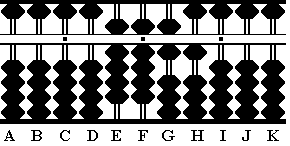Fig.6 `Step 1` ```A B C D E F G H I J K . . .     0 0 0 0 9 9 6 1 0 0 0 ```

Step 2: As seen above the complementary numbers in the subtrahend are 003. Therefore add 3 to rod H. This leaves 9964 on rods EFGH. (Fig.7)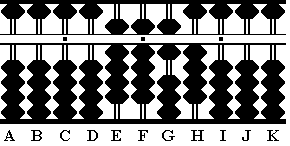Fig.7 `Step 2` ```A B C D E F G H I J K . . .     0 0 0 0 9 9 6 1 0 0 0 + 0 0 3 Step 2 0 0 0 0 9 9 6 4 0 0 0 ```

Step 3: Because there are 3 numbers in the subtrahend we need to subtract 1000 from rods EFGH.

3a & the answer: Add 1 to the result by adding 1 to rod H. This leaves the reminder 8965 on rods EFGH. Because rod F is the unit rod the answer actually reads 89.65 (Fig.8)Fig.8 `Step 3` ```A B C D E F G H I J K . . .     0 0 0 0 9 9 6 4 0 0 0 - 1 0 0 0 Step 3 0 0 0 0 8 9 6 4 0 0 0 + 1 Step 3a 0 0 0 0 8 9 6 5 0 0 0```

Fernando offers this observation:

If Edvaldo pays his debt of R\$89.65 using a R\$100.00 bill,
the amount of change due back to him can already be seen
on the soroban. No extra work is necessary. Once again it's
the ability to read complementary numbers that will provide

seen. Look at the complementary grey beads on rods EFGH.
Each of these is a complement of 9. They read 10.34. Mentally
add 1 to the complementary result to equal 10.35 and you have
the answer. If paying his debt with a R\$100.00 bill, the amount
due back to Edvaldo is R\$10.35Fig.8a

###The Negative Answer

Fernando's technique allows for a quick and easy calculation in the event that a subtraction problem requires subtracting a larger number from a smaller one resulting in a negative answer.

Example 3456 - 7324 = -3868

In this example the answer is a negative number. The technique used to solve problems of this type is a simplified version of the techniques used above in the first chapter, 'Simplifying Subtraction'.

Basically the method is this;

From the subtrahend, take the complementary numbers that make up 9.

Understanding the Complement

In looking at the subtrahend 7324, the complementary numbers that make up 9 will be 2675. Following Fernando's method, the number added to the minuend will be 2675. This will yield the correct negative answer.

The Solution

Step 1: Choose rod I to carry the unit number. Set 3456 on rods FGHI. (Fig.9)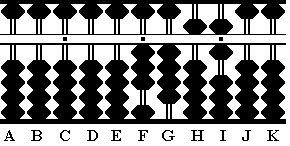Fig.9 ```A B C D E F G H I J K . . .     0 0 0 0 0 3 4 5 6 0 0 ```

Step 2: As seen above the complementary numbers for the subtrahend are 2675. Add this to the minuend. This leaves 6131 on rods FGHI.

2a: Read the negative answer in the form of the complementary numbers on the soroban. The negative numbers (the grey beads) reads -3868 on rods FGHI which is the correct answer. (Fig.10)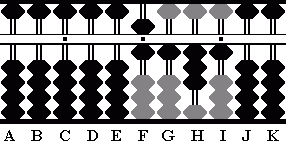Fig.10 ```A B C D E F G H I J K . . .     0 0 0 0 0 3 4 5 6 0 0 + 2 6 7 5 Step 2 0 0 0 0 0 6 1 3 1 0 0 ```

 REFERENCES: Tejón, Fernando Kojima, Takashi The Japanese Abacus, Its use and Theory Tokyo: Charles E. Tuttle, 1954 Print Page (.pdf format,140kb)

June, 2005
Fernando Tejón
Totton Heffelfinger